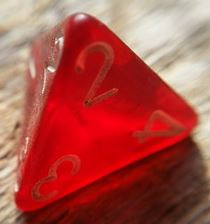# Using Manipulatives to Solve Probability Problems

Instructions:

question 1 of 3

Create Your Account To Take This Quiz

As a member, you'll also get unlimited access to over 79,000 lessons in math, English, science, history, and more. Plus, get practice tests, quizzes, and personalized coaching to help you succeed.

Try it risk-free for 30 days. Cancel anytime

### 2. How many total options does this probability manipulative have?Create your account to access this entire worksheet
Quizzes, practice exams & worksheets
Certificate of Completion
Create an account to get started

Our informative quiz/worksheet combo is a handy way to assess your knowledge of the use of manipulatives to solve probability problems. As you access these multiple-choice questions 24 hours a day, you'll be asked about using a quarter to find the probability of flipping heads or tails and how to answer example probability questions involving manipulatives.

## Quiz & Worksheet Goals

Take this quiz to see if you can answer questions on:

• The answer to a probability problem using a spinner
• Options for a pictured probability manipulative
• The probability of an outcome using dice
• Use of coins to determine probability

## Skills Practiced

• Interpreting information - verify that you can read information regarding the probability of flipping a head or a tail on a coin if you play 30 rounds and interpret it correctly
• Reading comprehension - ensure that you draw the most important information from the related lesson on using manipulatives to solve probability problems
• Problem solving - use acquired knowledge to solve probability practice problems

• Define probability as it's used in math
• Identify the classic game that utilizes a spinner
• Outline the formula to use when calculating the probability of throwing a number on a dice
Final Exam